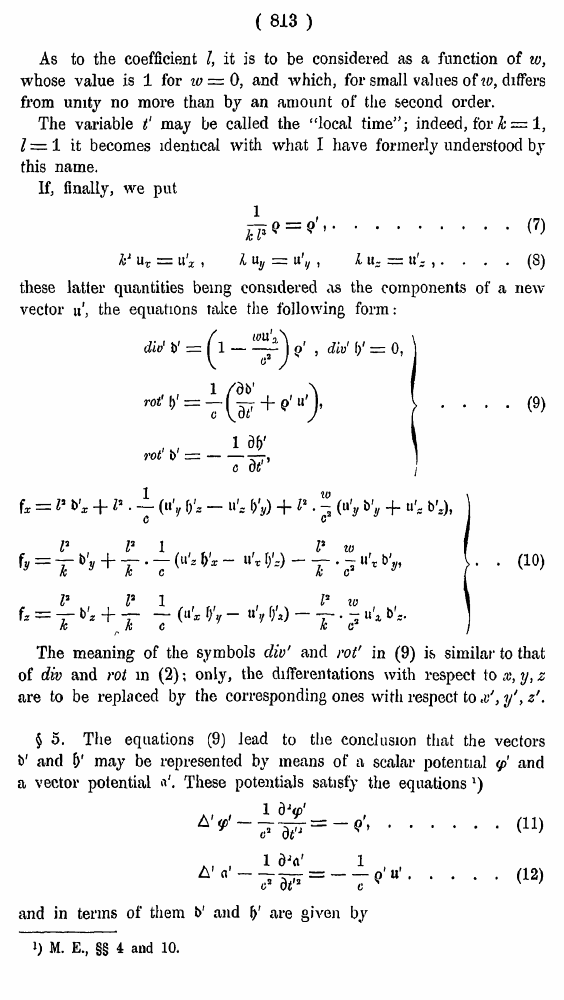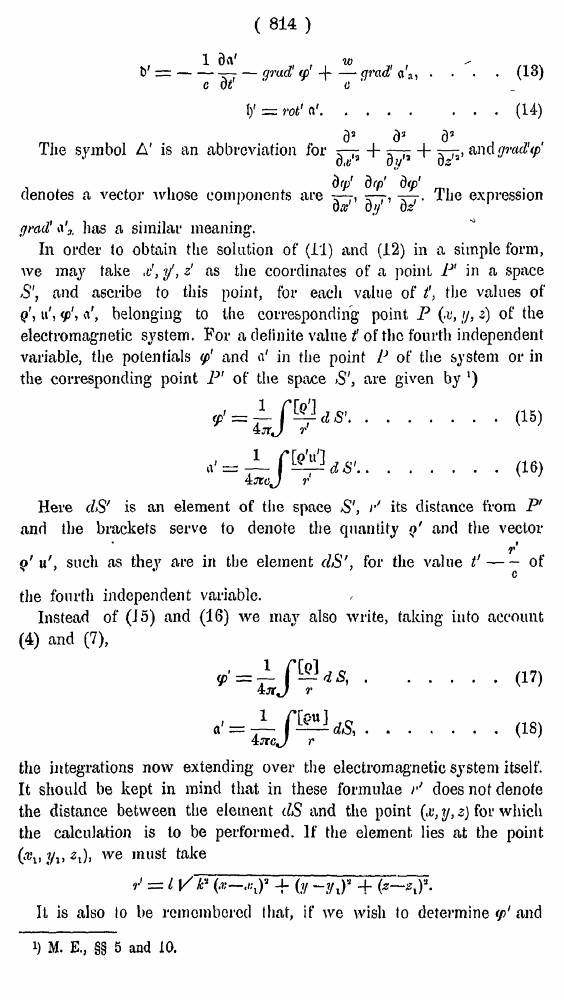# H. A. Lorentz. Electromagnetic phenomena in a system moving with any velocity smaller than that of light. // Proceedings Royal Acad., Amsterdam. Vol. VI., 1904

┬ эрірыю   ─Ёґушх ЄюЁьрҐ√   <<<     ╤ҐЁрэшІр 813   >>>809  810  811  812  813 814  815  816  817  818  819  820  821  822  823  824  825  826  827  828  829  830  831 ( 813 ) As to the coefficient I, it is to be considered as a function of w, whose value is 1 for 10 = 0, and which, for small values of to, differs from unity no more than by an amount of the second order. The variable tС may be called the Уlocal time5Т; indeed, for h = 1, Z = 1 it becomes identical with what I have formerly understood by this name. If, finally, we put ... (7) ... (8) these latter quantities being considered as the components of a new vector л', the equations take the following form : . . (9) . (10) The meaning of the symbols div' and rot' in (9) ivS similar to that of div and rot in (2); only, the differentations with respect to x, y, z are to be replaced by the corresponding ones with respect to y', z'. з 5. The equations (9) lead to the conclusion that the vectors b' and I)1 may be represented by means of a scalar potential ' and &' are given by 1) M. E., зз 4 and 10. ( 814 ) . . . (13) Х Х Х (14) The symbol A' is an abbreviation for, and gmdUp denotes a vector whose components areThe expression grail' has a similar meaning;. In order to obtain the solution of (11) and (12) in a simple form, we may take &, yС, z' as the coordinates of a point P1 in a space S', and ascribe to this point, for each value of ?, the values of q', u', \$', are given by ') ......(15) ......(16) Here clS' is an element of the space S', r' its distance from P' and the brackets serve to denote the quantity ;'A> 2i)i we must take It is also lo be remembered that, if we wish to determine